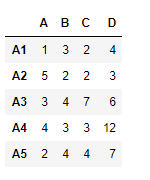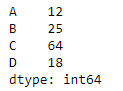Related Articles

# Python | Pandas dataframe.sub()

• Difficulty Level : Expert
• Last Updated : 14 Sep, 2021

Python is a great language for doing data analysis, primarily because of the fantastic ecosystem of data-centric python packages. Pandas is one of those packages and makes importing and analyzing data much easier.
Pandas dataframe.sub() function is used for finding the subtraction of dataframe and other, element-wise. This function is essentially same as doing dataframe – other but with support to substitute for missing data in one of the inputs.

Syntax: DataFrame.sub(other, axis=’columns’, level=None, fill_value=None)
Parameters :
other : Series, DataFrame, or constant
axis : For Series input, axis to match Series index on
level : Broadcast across a level, matching Index values on the passed MultiIndex level
fill_value : Fill existing missing (NaN) values, and any new element needed for successful DataFrame alignment, with this value before computation. If data in both corresponding DataFrame locations is missing the result will be missing.
Returns : result : DataFrame

Example #1: Use sub() function to subtract each element of a dataframe with a corresponding element in a series.

## Python3

 `# importing pandas as pd``import` `pandas as pd` `# Creating the dataframe``df ``=` `pd.DataFrame({``"A"``:[``1``, ``5``, ``3``, ``4``, ``2``],``                   ``"B"``:[``3``, ``2``, ``4``, ``3``, ``4``],``                   ``"C"``:[``2``, ``2``, ``7``, ``3``, ``4``],``                   ``"D"``:[``4``, ``3``, ``6``, ``12``, ``7``]},``                   ``index ``=``[``"A1"``, ``"A2"``, ``"A3"``, ``"A4"``, ``"A5"``])` `# Print the dataframe``df`Let’s create the series

## Python3

 `# importing pandas as pd``import` `pandas as pd` `# Create the series``sr ``=` `pd.Series([``12``, ``25``, ``64``, ``18``], index ``=``[``"A"``, ``"B"``, ``"C"``, ``"D"``])` `# Print the series``sr`Let’s use the dataframe.sub() function for subtraction.

## Python3

 `# equivalent to df - sr``df.sub(sr, axis ``=` `1``)`

Output :Example #2: Use sub() function to subtract each element in a dataframe with the corresponding element in other dataframe

## Python3

 `# importing pandas as pd``import` `pandas as pd` `# Creating the first dataframe``df1 ``=` `pd.DataFrame({``"A"``:[``1``, ``5``, ``3``, ``4``, ``2``],``                    ``"B"``:[``3``, ``2``, ``4``, ``3``, ``4``],``                    ``"C"``:[``2``, ``2``, ``7``, ``3``, ``4``],``                    ``"D"``:[``4``, ``3``, ``6``, ``12``, ``7``]},``                    ``index ``=``[``"A1"``, ``"A2"``, ``"A3"``, ``"A4"``, ``"A5"``])` `# Creating the second dataframe``df2 ``=` `pd.DataFrame({``"A"``:[``10``, ``11``, ``7``, ``8``, ``5``],``                    ``"B"``:[``21``, ``5``, ``32``, ``4``, ``6``],``                    ``"C"``:[``11``, ``21``, ``23``, ``7``, ``9``],``                    ``"D"``:[``1``, ``5``, ``3``, ``8``, ``6``]},``                    ``index ``=``[``"A1"``, ``"A2"``, ``"A3"``, ``"A4"``, ``"A5"``])` `# subtract df2 from df1``df1.sub(df2)`

Output :Notice, each element of the dataframe df1 has been subtracted with the corresponding element in the df2.

Attention geek! Strengthen your foundations with the Python Programming Foundation Course and learn the basics.

To begin with, your interview preparations Enhance your Data Structures concepts with the Python DS Course. And to begin with your Machine Learning Journey, join the Machine Learning – Basic Level Course

My Personal Notes arrow_drop_up